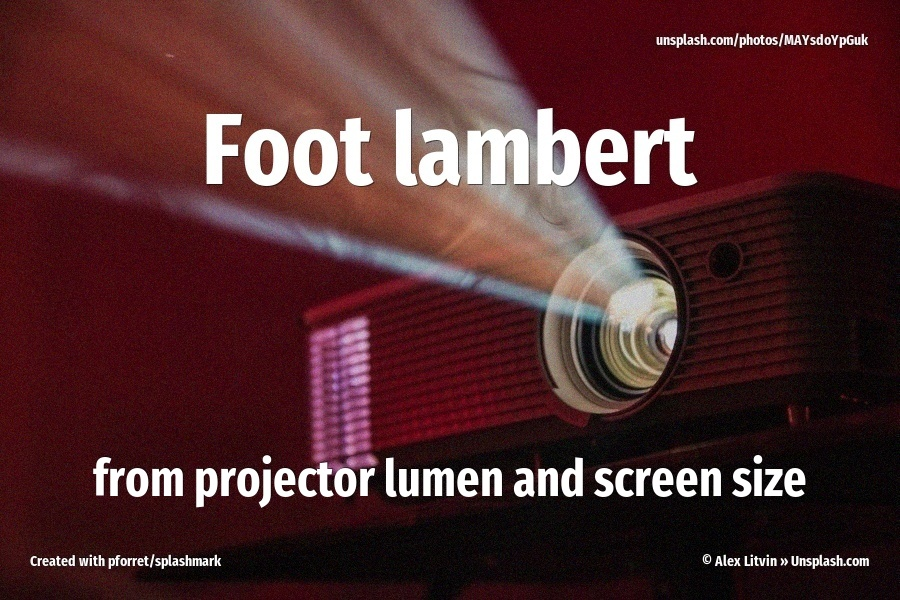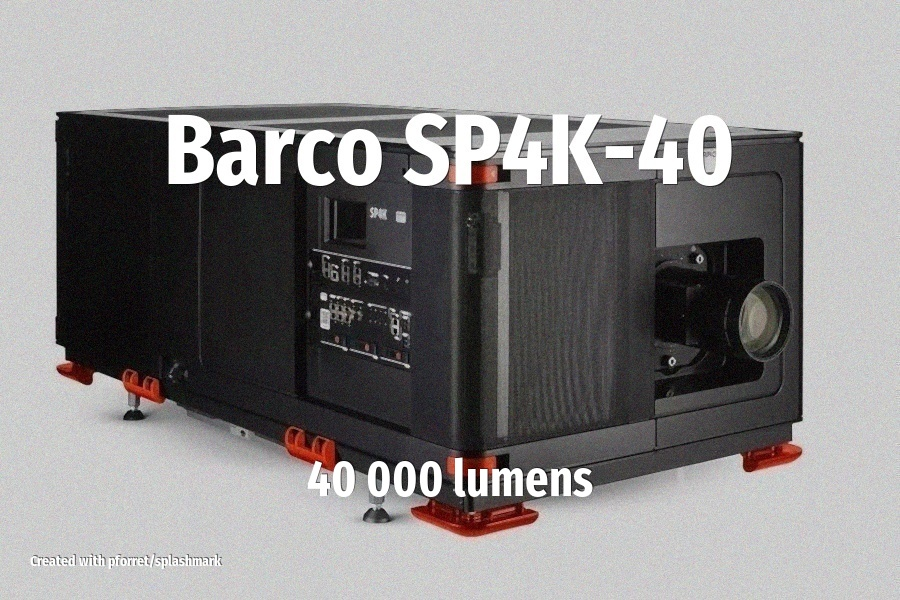# Calculate foot-lambert from screen size, projector lumen

In the projection business, there is a unit for ‘how much light is coming from that screen, actually?’. This is called the foot-Lambert or fL.

To calculate foot lamberts (fL) from lumens and screen size, you can use the following formula:

Foot Lamberts (fL) = Lumens / (Screen Area in square feet)Here’s how you can calculate it correctly:

Determine the screen area in square feet.

Screen Area (square feet) = (Screen Width in feet) × (Screen Height in feet)

Calculate the foot lamberts using the formula mentioned above:

Foot Lamberts (fL) = Lumens / Screen Area

Let’s use an example to illustrate this:Suppose you have a cinema projector with a brightness of 40 000 lumens (e.g. the above Barco SP4K-40). The screen is 23 meters wide, and has the Cinema Scope (2.35:1) aspect ratio.

Let’s calculate the screen area in square feet. If the width is 23 meters and the aspect ratio is 2.35:1, we can calculate the height using the aspect ratio formula:

Screen Height = Screen Width / Aspect Ratio = 23 meters / 2.35 = 9.79 meters

converted to imperial units: the screen is 75.5 feet wide and 32.1 feet high

So the screen area in square feet

= 75.5 feet × 32.1 feet = 2424 square feet.

Calculate the foot lamberts:

Foot Lamberts (fL) = 40000 lumens / 2424 square feet = 16.5 fL foot lambert